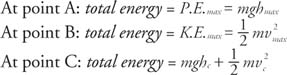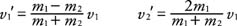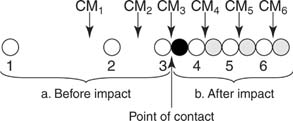## Work and Energy

The concepts of work and energy are closely tied to the concept of force because an applied force can do work on an object and cause a change in energy. Energy is defined as the ability to do work.

## Work

The concept of work in physics is much more narrowly defined than the common use of the word. Work is done on an object when an applied force moves it through a distance. In our everyday language, work is related to expenditure of muscular effort, but this is not the case in the language of physics. A person that holds a heavy object does no physical work because the force is not moving the object through a distance. Work, according to the physics definition, is being accomplished while the heavy object is being lifted but not while the object is stationary. Another example of the absence of work is a mass on the end of a string rotating in a horizontal circle on a frictionless surface. The centripetal force is directed toward the center of the circle and, therefore, is not moving the object through a distance; that is, the force is not in the direction of motion of the object. (However, work was done to set the mass in motion.) Mathematically, work is W = F · x, where F is the applied force and x is the distance moved, that is, displacement. Work is a scalar. The SI unit for work is the joule (J), which is newton‐meter or kg m/s 2.

If work is done by a varying force, the above equation cannot be used. Figure shows the force‐versus‐displacement graph for an object that has three different successive forces acting on it. The force is increasing in segment I, is constant in segment II, and is decreasing in segment III. The work performed on the object by each force is the area between the curve and the x axis. The total work done is the total area between the curve and the x axis. For example, in this case, the work done by the three successive forces is shown in Figure 1.Figure 1

Acting force changing with position.

In this example, the total work accomplished is (1/2)(15)(3) + (15)(2) + (1/2)(15)(2) = 22.5 + 30 + 15; work = 67.5 J. For a gradually changing force, the work is expressed in integral form, W = ∫ F · dx.

## Kinetic energy

Kinetic energy is the energy of an object in motion. The expression for kinetic energy can be derived from the definition for work and from kinematic relationships. Consider a force applied parallel to the surface that moves an object with constant acceleration.

From the definition of work, from Newton's second law of motion, and from kinematics, W = Fx = max and v f 2 = v o 2 + 2 ax, or a = ( v f 2v o 2)/2 x. Substitute the last expression for acceleration into the expression for work to obtain W = m ( v f 2v o 2) or W = (1/2) mv f 2 − (1/2) mv o 2. The right side of the last equation yields the definition for kinetic energy: K. E. = (1/2) mv 2 Kinetic energy is a scalar quantity with the same units as work, joules (J). For example, a 2 kg mass moving with a speed of 3 m/s has a kinetic energy of 9 J.

The above derivation shows that the net work is equal to the change in kinetic energy. This relationship is called the work‐energy theorem: W net = K. E. f K. E. o , where K. E. f is the final kinetic energy and K. E. o is the original kinetic energy.

## Potential energy

Potential energy, also referred to as stored energy, is the ability of a system to do work due to its position or internal structure. Examples are energy stored in a pile driver at the top of its path or energy stored in a coiled spring. Potential energy is measured in units of joules.

Gravitational potential energy is energy of position. First, consider gravitational potential energy near the surface of the earth where the acceleration due to gravity (g) is approximately constant. In this case, an object's gravitational potential energy with respect to some reference level is P.E. = mgh, where h is the vertical distance above the reference level. To lift an object slowly, a force equal to its weight (mg) is applied through a height (h). The work accomplished is equal to the change in potential energy: W = P. E. f P. E. o = mgh f mgh o , where the subscripts (f and o) refer to the final and original heights of the body.

Launching a rocket into space requires work to separate the mass of the earth and the rocket to overcome the gravitational force. For large distances from the center of the earth, the above equation is inadequate because g is not constant. The general form of gravitational potential energy is P.E. = − GMm/r, where M and m refer to the masses of the two bodies being separated and r is the distance between the centers of the masses. The negative sign is a result of selecting the zero reference at r equal to infinity, that is, at very large separation.

Elastic potential energy is energy stored in a spring. The magnitude of the force required to stretch a spring is given by F = − kx, where x is the distance of stretch (or compression) of a spring from the unstressed position, and k is the spring constant. The spring constant is a measure of the stiffness of the spring, with stiffer springs having larger k values. The potential energy stored in a spring is given by P. E. = (1/2) kx 2.

Change in potential energy is equal to work. The gravitational force and the force to stretch a spring are varying forces; therefore, the potential energy equations given above for these two cases can also be derived from the integral form of work, Δ P. E. = W = ∫ F · dx.

## Power

Power is the rate of doing work, average P = W/t, where t is the time interval during which work (W) is accomplished. Another form of power is found from W = F Δ x and substitution of average velocity of the object during time t for Δ x/ t: average P = F Δ xt = F(average v).

## The conservation of energy

The principle of conservation of energy is one of the most far‐reaching general laws of physics. It states that energy is neither created nor destroyed but can only be transformed from one form to another in an isolated system.

Because the total energy of the system always remains constant, the law of conservation of energy is a useful tool for analyzing a physical situation where energy is changing form. Imagine a swinging pendulum with negligible frictional forces. At the top of its rise, all the energy is gravitational potential energy due to height above the stationary position. At the bottom of the swing, all the energy has been transformed into kinetic energy of motion. The total energy is the sum of the kinetic and potential energies. It maintains the same value throughout the back and forth motion of a swing (see Figure 2).Figure 2

A pendulum obeys the law of conservation of energy.At point C, the potential energy is dependent upon the height, and the rest of the total energy is kinetic energy.

Although total energy is conserved, kinetic energy need not be conserved. A collision between two objects with conservation of kinetic energy is called an elastic collision. Colliding objects interacting with losses of kinetic energy due to frictional losses or deformation of an object are called inelastic collisions. In the macroscopic world, most collisions are inelastic; however, losses of kinetic energy are negligible in the nearly elastic collisions between atomic particles and subatomic particles. For these cases, the law of conservation of momentum and the conservation of kinetic energy yield useful equations.

Consider a simple head‐on elastic collision where one mass ( m 1) with a given velocity ( v 1) hits a second mass ( m 2) that is initially at rest. Apply the laws of conservation of momentum and conservation of kinetic energy to get m 1 v 1 = m 1v 1 + m 2 v 2′ and (1/2) m 1 v 1 2 = (1/2) m 1 v 12 + (1/2) m 2 v2 2, where the primes refer to velocities after the collision. Solving the equations gives the velocities of the two masses after the interaction:Three special cases are instructive:

For equal masses where m 1 = m 2, note that v 1′ becomes zero and v 2′ equals v 1; thus, for equal masses, the objects simply exchange velocities, as is sometimes observed with pool balls. (Pool balls have rotational energy and somewhat inelastic collisions, so their behavior only approximates the example.)

If m 2 is massive, the numerator and denominator are nearly the same in the equation for v 1′. Then, v 1′ is approximately equal to v 1 but in the opposite direction. The denominator of the expression for v 2′ will be so large so that the velocity of the second mass after the collision will be small. In other words, the incoming mass ( m 1) will bounce back off the second mass with nearly the initial speed, and the hit mass ( m 2) will move slowly after the collision.

If m 1 is massive, then v 1′ is approximately equal to v 1, and v 2′ is nearly twice v 1; or the incoming massive particle continues at nearly the same velocity, and the hit mass moves ahead at nearly twice the initial velocity of the first mass after the collision.

## Center of mass

The concept of the center of mass (CM) is useful to analyze the motion of a system of particles. The system of particles acts as if all of its mass is concentrated at the CM. In the absence of an external force, if the CM of the system is at rest, then it will remain at rest, and if it is initially in motion, it will maintain that motion. In other words, the CM moves in accordance with Newton's second law. The x and y coordinates of the center of mass areConsider the previous example of a head‐on collision of two equal masses that stick together after the collision. The CM is initially in motion at a constant velocity and maintains the same velocity after the collision. As the first mass rolls in toward the second mass, the CM is always halfway between the two masses. Before the collision, the CM covers one‐half the distance of the incoming object in the same time, and therefore, the velocity of the CM is one‐half the initial velocity of the incoming mass. For the instant that the two masses interact, the CM is right between the two objects. After the collision, the masses stick together and have one‐half the initial velocity because the effective mass has doubled. The CM continues midway between the masses. It maintains the same velocity of (1/2) v o after the collision. In Figure , the moving white ball impacts the stationary black ball. The numbered and circled positions of the CM correspond to the numbered positions of the balls.Figure 3

Inelastic collision of two balls.